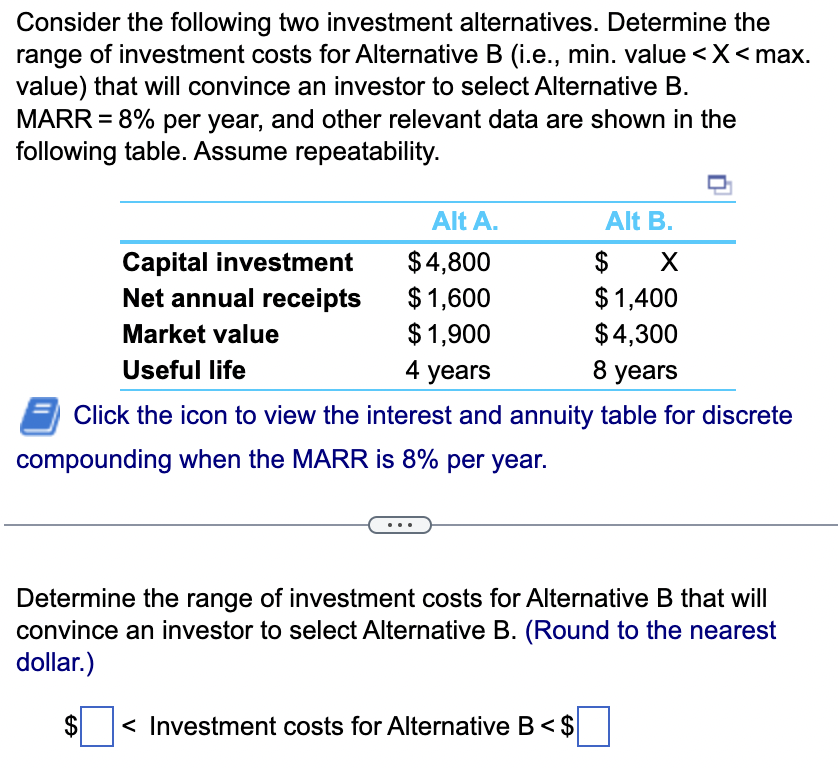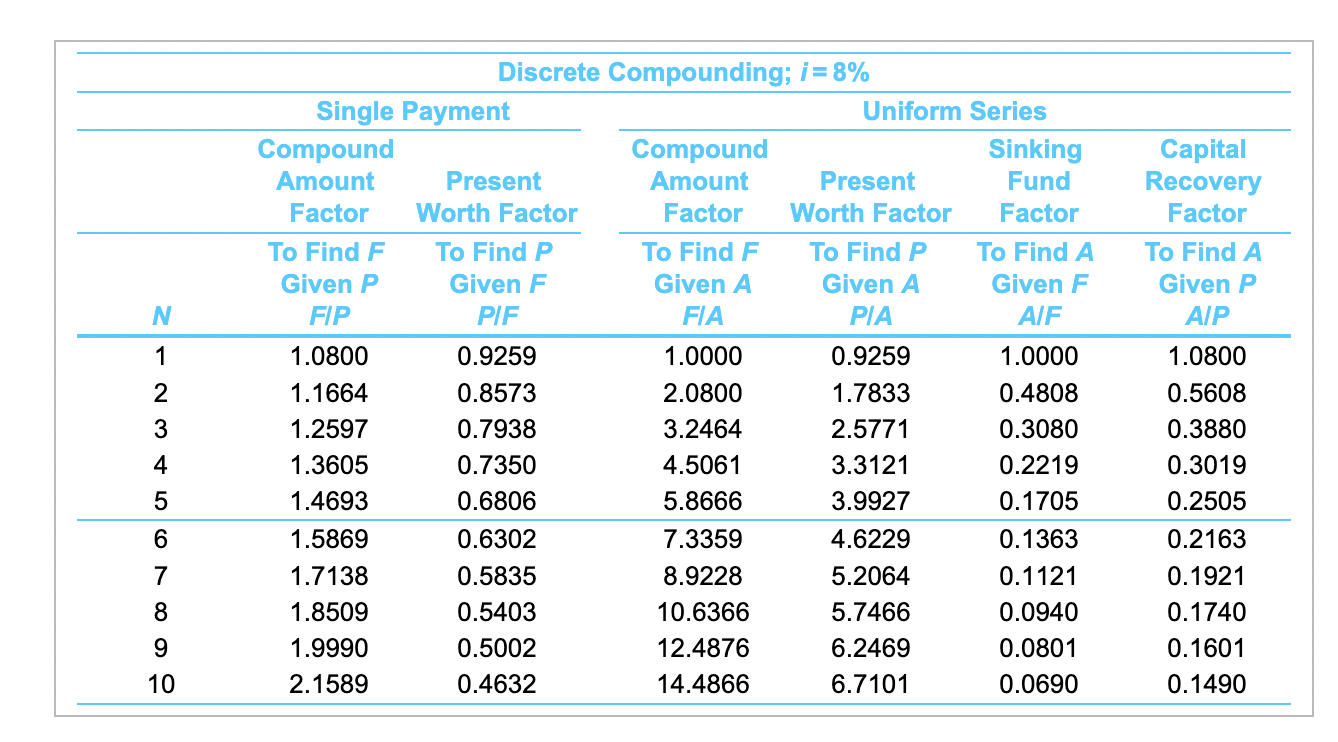Home / Expert Answers / Operations Management / consider-the-following-two-investment-alternatives-determine-the-range-of-inve-pa301

# (Solved): Consider the following two investment alternatives. Determine the range of inve ...???????Consider the following two investment alternatives. Determine the range of investment costs for Alternative B? (i.e., min. valueXmax. ?value) that will convince an investor to select Alternative B. MARR?% per? year, and other relevant data are shown in the following table. Assume repeatability. Alt A. Alt B. Capital investment ?\$ ?\$ X Net annual receipts ?\$ ?\$ Market value ?\$ ?\$ Useful life years years LOADING... Click the icon to view the interest and annuity table for discrete compounding when the MARR is ?% per year. Question content area bottom Part 1 Determine the range of investment costs for Alternative B that will convince an investor to select Alternative B. ?(Round to the nearest? dollar.) ?\$ ?? enter your response here Investment costs for Alternative B?\$ ?? enter your response here

Consider the following two investment alternatives. Determine the range of investment costs for Alternative \( B \) (i.e., min. value \(

We have an Answer from Expert